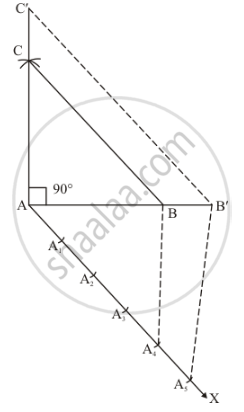Share

# Draw a Right Triangle Abc In Which Ac = Ab = 4.5 Cm and ∠A = 90°. Draw a Triangle Similar to δAbc With Its Sides Equal to (5/4)Th of the Corresponding Sides of δAbc. - CBSE Class 10 - Mathematics

#### Question

Draw a right triangle ABC in which AC = AB = 4.5 cm and ∠A = 90°. Draw a triangle similar to ΔABC with its sides equal to (5/4)th of the corresponding sides of ΔABC.

#### Solution

Given that

Construct a right triangle of sides AC = AB = 4.5 cm and ∠A = 90° and then a triangle similar to it whose sides are (5/4)th of the corresponding sides of ΔABC.

We follow the following steps to construct the givenStep of construction

Step: I- First of all we draw a line segment AB = 4.5cm.

Step: II- With as centre and draw an angle ∠A = 90°.

Step: III- With as centre and radius AC = 4.5 cm.

Step: IV- Join BC to obtain ΔABC.

Step: V- Below AB, makes an acute angle ∠BAX = 60°.

Step: VI- Along AX, mark off five points A1, A2, A3, A4 and A5, such that AA1 = A1A2 = A2A3 = A3A4 = A4A5

Step: VII-Join A4B.

Step: VIII- Since we have to construct a triangle each of whose sides is (5/4)th of the corresponding sides of ΔABC.

So, we draw a line A5B' on AX from point A5 which is A5B' || A4B, and meeting AB at B’.

Step: IX- From B’ point draw B'C'||BC and meeting AC at C’

Thus, ΔAB'C' is the required triangle, each of whose sides is (5/4)th of the corresponding sides of ΔABC.

Is there an error in this question or solution?

#### APPEARS IN

Solution Draw a Right Triangle Abc In Which Ac = Ab = 4.5 Cm and ∠A = 90°. Draw a Triangle Similar to δAbc With Its Sides Equal to (5/4)Th of the Corresponding Sides of δAbc. Concept: Division of a Line Segment.
S